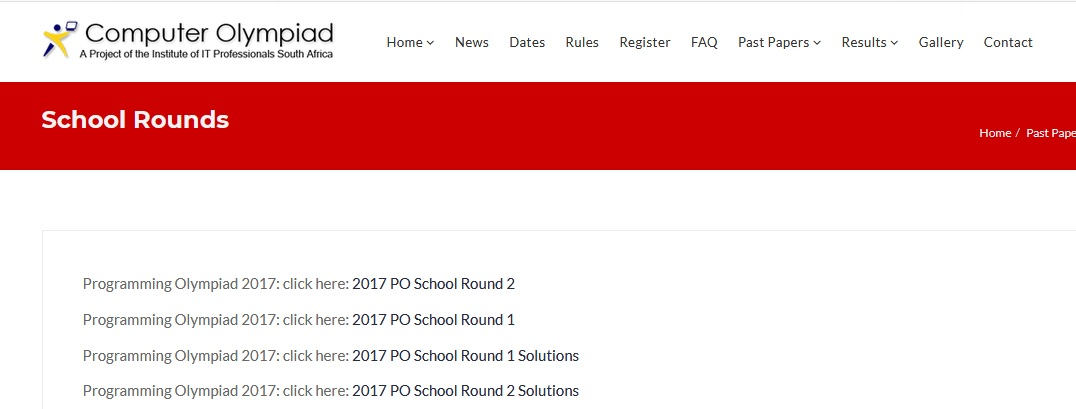1. Home
2. /
3. Question Paper
4. /
All posts from

# Computer Programming Olympiad Round 1 Question Paper 2017

Name of the Organization : Computer Olympiad South Africa
Announcement : Programming Olympiad Round 1 Question Paper
Year : 2017
Type : Past Papers
2017 PO School Round 1 : https://www.southafricain.com/uploads/9860-2017POR1.pdf
2016 PO School Round 1 : https://www.southafricain.com/uploads/9860-2016POR1.pdf
2015 PO School Round 1 : https://www.southafricain.com/uploads/9860-2015POR1.pdf
2014 PO School Round 1 : https://www.southafricain.com/uploads/9860-2014POR1.pdf
2013 PO School Round 1 : https://www.southafricain.com/uploads/9860-2013POR1.pdf
2012 PO School Round 1 : https://www.southafricain.com/uploads/9860-2012POR1.pdf
2011 PO School Round 1 : https://www.southafricain.com/uploads/9860-2011POR1.pdf

Want to comment on this post?

## Computer Programming Olympiad Round 1 Question Paper

** Not to be used before 14 August 2017

Related : Computer Programming Olympiad Contest 2018 : www.southafricain.com/9817.html

## Instructions

1. This paper is for ALL candidates.

3. Each correct answer earns 10 marks.
4. You have 60 minutes to attempt as many questions as possible.
5. Programs should be readable, concise, and use appropriate variable names.

6. Indicate the question, your name, surname and the language and version used at the start of every program e.g. “Q3 Sam King, Python 2.7”
7. Save your program as Qn Name Surname e.g. Q3 Sam King

8. You may assume that the user input will satisfy the problem specification and so you do not need to validate the input.
9. Do not write code to produce only specific answers, as the external judges may use other test cases.

10. After the contest you may be given time to print out your Answer Sheet. Do not make any changes.11. Make sure you save the programs you have created and the Answer Sheet in a place where your teacher can find them.

12. Do Not Modify Any Files After The End Of The Contest As This Will Lead To Disqualification.

## PO Sample Questions

Section – 1 : Simple Interest
Section – 2 : Tribonacci
Section – 3 : Reading Level

### Simple Interest

If you invest using simple interest, the amount you get back is calculated using the following formula:
where r is the interest rate (as a percentage from 0 to 100), P is the initial investment, and t is the number of years of the investment. The answer A is the amount after t years. Task: Write a program that takes the interest rate, the number of years and the initial investment, and then computes what your investment would amount to after t years. The output value of A should be to exactly two decimal places.

Examples :
Input: P = 5000 r = 12 t = 5
Output: A = 8000.00
Input: P = 3750 r = 7.5 t = 10
Output: A = 6562.50
Test your program with the following cases and type or paste the answers in the correct blocks on the answer sheet.
a) P = 150 r = 6 t = 10
b) P = 10000 r = 6.75 t = 5.5

### Tribonacci

The Fibonacci sequence is a well-known sequence of integers defined by the following rule. The first number (term) is 0, the second number (term) is 1, and each number (term) after that is defined by adding the two previous terms in the sequence together.

The Fibonacci sequence therefore goes as follows :
0, 1, 1, 2, 3, 5, 8, 13, 21, 34, 55, …
We are interested in a generalisation of the Fibonacci sequence called the Tribonnaci sequence. This sequence is generated by starting with 3 given integers and then each successive number is formed by adding the previous 3 terms together.

For example, if we start with the integers 1, 2 and 3, the Tribonacci sequence will be as follows:
1, 2, 3, 6, 11, 20, 37, 68, 125, 230, 423, 778, …

Task : Create a program that will produce the nth term of a Tribonacci sequence, given the three starting integers.
Examples :

Test your program with the following cases and type or paste the answers in the correct blocks on the web page:

A simple way of checking the reading level of a given piece of text is to determine the average length of the words in the text.

Task : Write a program that will calculate the average length of the words for any given piece of text and give the answer rounded off to two decimal places. Only the 26 letters of the alphabet (upper and lower case) must be taken into consideration. The given text may include other punctuation marks which should NOT be considered part of the text.

Examples:
Input: How now brown cow?
Output: 3.50
Input: “Stay inside the house”, she said.
Output: 4.17

Test your program with the following cases and type or paste the answers in the correct blocks on the web page:
a) Mary had a little lamb
b) Upon this followed irritation, aggravation and frustration.
c) Most newspapers — and all that follow AP style — insert a space before and after the em dash.
d) “Stop!”, shouted Chris. He said to Emily, “Why were you running in the hall?”